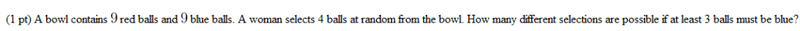# What's wrong with my logic on this combinatorial/probability question?

jdinatale

## Homework Statement## The Attempt at a Solution

If at least 3 balls must be blue, then the possible ways of selecting at least 3 blues are:

r, b, b, b
b, r, b, b
b, b, r, b
b, b, b, r
b, b, b, b

where r = red ball, and b = blue ball

So there are 5 ways to draw 3+ blue.